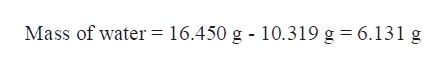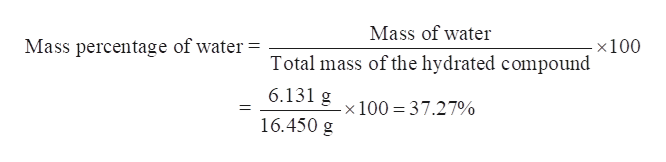A sample of an unknown hydrated compound weighed 16.450 grams. After heating, it had a mass of 10.319 grams. Calculate the percentage of water by mass in the unknown.

Question

A sample of an unknown hydrated compound weighed 16.450 grams. After heating, it had a mass of 10.319 grams. Calculate the percentage of water by mass in the unknown.

Step 1

We have to calculate the percentage of water by mass in the unknown hydrated compound that weighed 16.450 grams. After heating, sample had a mass of 10.319 grams.

Step 2

Calculate the mass of water in the sample of unknown hydrated compound-

On heating of hydrated compound, it loses all its water molecules.

Thus, Mass of water in sample = mass of hydrated compound – mass of heated compound.help_outlineImage TranscriptioncloseMass of water= 16.450 g - 10.319 g = 6.131 g fullscreen
Step 3

Therefore, mass of water = 6.131 g.

Now calculate the mass percenta...help_outlineImage TranscriptioncloseMass of water Mass percentage of water x100 Total mass of the hydrated compound 6.131 g 100 37.27% 16.450 g fullscreen

Want to see the full answer?

See Solution

Want to see this answer and more?

Our solutions are written by experts, many with advanced degrees, and available 24/7

See Solution
Tagged in

General Chemistry